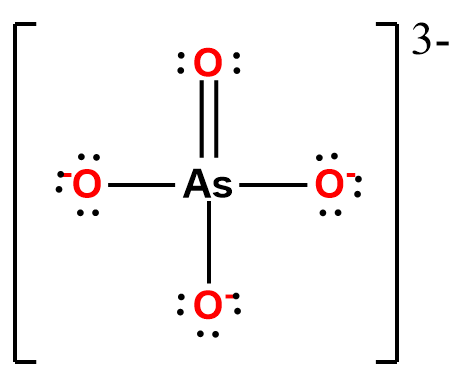# Problem: Draw the Lewis structure of ASO43- showing all lone pairs. What is the hybridization of the central As atom? a) spb) sp2c) sp3d) sp3de) sp3d2An AsO43- ion is a) nonpolarb) polarIdentify the molecular geometry of AsO43-. a) linearb) see-sawc) square pyramidald) trigonal planare) t-shapedf) square planarg) benth) trigonal bipyramidali) trigonal pyramidalj) tetrahedralk) octahedralWhat are the approximate bond angles in ASO43-?a) 90 degreesb) 109 degreesc) 120 degreesd) 180 degrees

###### FREE Expert Solution

Step 1: Arsenic (EN = 2.18) is less electronegative than oxygen (3.44) so arsenic is the central atom.

Step 2: The total number of valence electrons present in AsO43- is:

Group             Valence Electrons

As       5A                   1 × 5 e5 e

O         6A                   4 × 6 e24 e

From -3 charge:                         +3e-

Total:  32 valence e

Step 3: The Lewis Structure is:Step 4: There are 4 domains or groups around central element As:

▪ 4 O atoms = 4 domains

• It will need 4 orbitals to accommodate these 4 groups.

Orbitals that can be used by the central atom:

Subshell                   Total # of orbitals

s             _                 1 orbital

p          _ _ _              3 orbitals

d        _ _ _ _ _          5 orbitals

1 s orbital + 3 p orbitals = 4 orbitals (4 groups)

s p p p = sp3

90% (148 ratings)###### Problem Details

Draw the Lewis structure of ASO43- showing all lone pairs.

What is the hybridization of the central As atom?

a) sp

b) sp2

c) sp3

d) sp3d

e) sp3d2

An AsO43- ion is

a) nonpolar

b) polar

Identify the molecular geometry of AsO43-

a) linear

b) see-saw

c) square pyramidal

d) trigonal planar

e) t-shaped

f) square planar

g) bent

h) trigonal bipyramidal

i) trigonal pyramidal

j) tetrahedral

k) octahedral

What are the approximate bond angles in ASO43-?

a) 90 degrees

b) 109 degrees

c) 120 degrees

d) 180 degrees